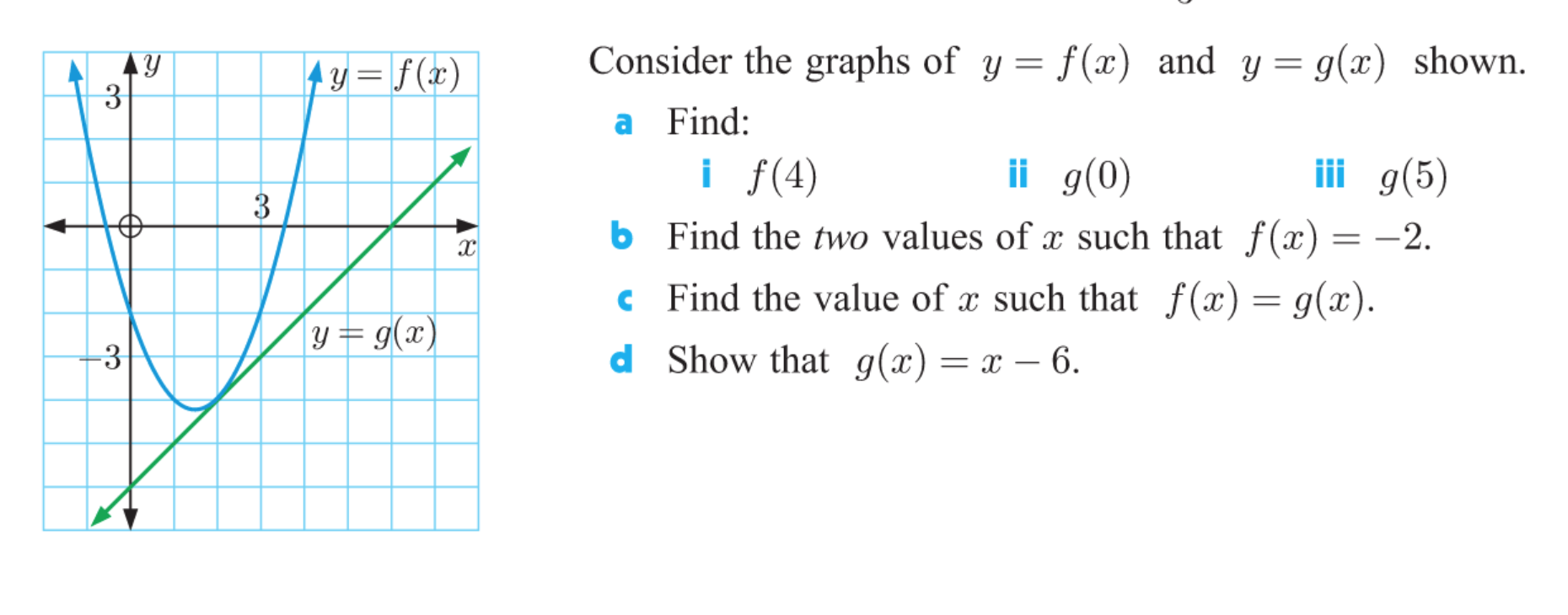# Consider the graphs of y = f(x) and y= g(x) shown.4y= f (x)3.a Find:ii g(0)i f(4)6 Find the two values of x such that f(x) =iii g(5)-2.c Find the value of a such that f(x) = g(x).y= g(x)d Show that g(x)= x – 6.-33.

Question
16 viewshelp_outlineImage TranscriptioncloseConsider the graphs of y = f(x) and y= g(x) shown. 4y= f (x) 3. a Find: ii g(0) i f(4) 6 Find the two values of x such that f(x) = iii g(5) -2. c Find the value of a such that f(x) = g(x). y= g(x) d Show that g(x)= x – 6. -3 3. fullscreen
check_circle

Step 1

Since we only answer up to 3 sub-parts, we’ll answer the first 3. Please resubmit the question and specify the other subparts (up to 3) you’d like answered.

a) i) For f(4) we have to find f(x) at x=4. f(x) is the blue graph with (4,2) on it. At x=4 , f(x)= 2. So f(4)=2

ii) For g(0) we have to find g(x) at x=0. g(x) is the green graph with (0,-6) on it. At x=0 , g(x)= -6 . So g(0)=-6

iii) For g(5) we have to find g(x) at x=5. g(x) is the green graph with (5,-1) on it. At x=5 , g(x)= -1 . So g(5)=-1

Answer(a): f(4)=2 , g(0)=-6 , g(5)=-1

Step 2

b) Given f(x)=-2 so we draw y=-2 line and check where it cuts f(x).

It cuts at (0,...

### Want to see the full answer?

See Solution

#### Want to see this answer and more?

Solutions are written by subject experts who are available 24/7. Questions are typically answered within 1 hour.*

See Solution
*Response times may vary by subject and question.
Tagged in